# Ncert Class 6 Math Integers Exercise 6.2

Integers Class 6 Ex. 6.2

New Ncert Class 6 Math Free Solution.

Exercise 6.2

Question 1 :- Using the number line write the integers which is:
(a) 3 more than 5
(b) 5 more than -5
(c) 6 less than 2
(d) 3 less than -2

Solution 1:-

(a) 3 more than 5
From 5, we take 3 steps to the right to get to 8.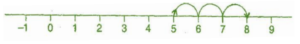Hence, 3 more than 5 = 8.

(b) 5 more than -5
From -5, we take 5 steps to the right to get to 0.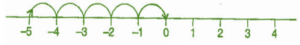Hence, 5 more than -5 = 0.

(c) 6 less than 2
From 2, we take 6 steps to the left to get to – 4.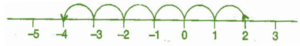Hence, 6 less than 2 = -4.

(d) 3 less than -2
From – 2, we take 3 steps to the left to get to – 5.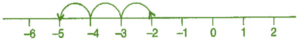Hence, 3 less than -2 = -5.

Question 2 :- Use number line and add the following integer:
(a) 9 + (-6)
(b) 5 + (-11)
(c) (-1) + (-7)
(d) (- 5) + 10
(e) (-1) + (-2) + (-3)

Solution 2:-

(a) 9 + (- 6) = 3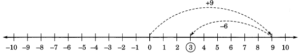(b) 5 + (-11) = -6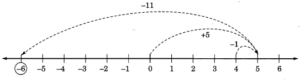(c) (-1) + (-7) = -8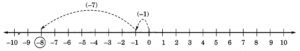(d) (-5) + 10 = 5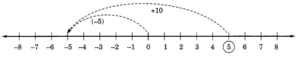(e) (-1) + (-2) + (-3) = -6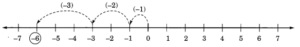(f) (-2) + 8 + (-4) = 2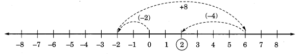Question 3 :- Add without using number line:
(а) 11 + (-7)
(b) (-13) + (+18)
(c) (-10) + (+19)
(d) (-250) + (+150)
(e) (-380) + (-270)
(f) (-217) + (-100).

Solution 3:-

(a) 11 + (-7)
= 11 – 7
=  4
Hence, 11 + (-7) = 4.

(b) (-13) + (+18)
=  – 13 + 18
= 5
Hence, (- 13) + (+ 18) = 5.

(c) (-10) + (+19)
=  – 10 + 19
= 9
Hence, (-10) + (19) = 9.

(d) (-250) + (+150)
= – 250 + 150
= -100
Hence, (-250) + (+150) = – 100.

(e) (-380) + (-270)
= – 380 – 270
= – 650
Hence, (-380) + (-270) = (-650).

(f) (-217) + (-100)
= -217 -100
= -317
Hence, (-217) + (-100) = -317

Question 4 :- Find the sum of:
(a) 137 and -354
(b) -52 and 52 .
(d) -312, 39 and 192
(d) -50, -200 and 300

Solution 4:-

(a) 137 and -354
(137) + ( -354)
= 137 -137
= 0

(b) -52 and 52
(-52) + (+52)
= -52 + 52
= 0

(c) -312,  39  and 192
(-312) + (+ 39) + (+192)
= – 312 + 39 + 192
= – 312 + 231
= – 81

(d) – 50, -200 and 300
(-50) + (-200) + (+ 300)
= -50  -200 + 300
= – 250 + 300
= 50

Question 5 :- Find the sum :
(a) (- 7) + (-9) + 4 + 16
(b) (37) + (-2) + (-65) + (-8)

Solution 5:-

(a) (- 7) + (-9) + 4 + 16
= -7  -9  +  4  + 16
= – 16 + 20
= 4

(b) (37) + (-2) + (-65) + (-8)
= +37 – 2 – 65 – 8
= +37 – 75
= -38